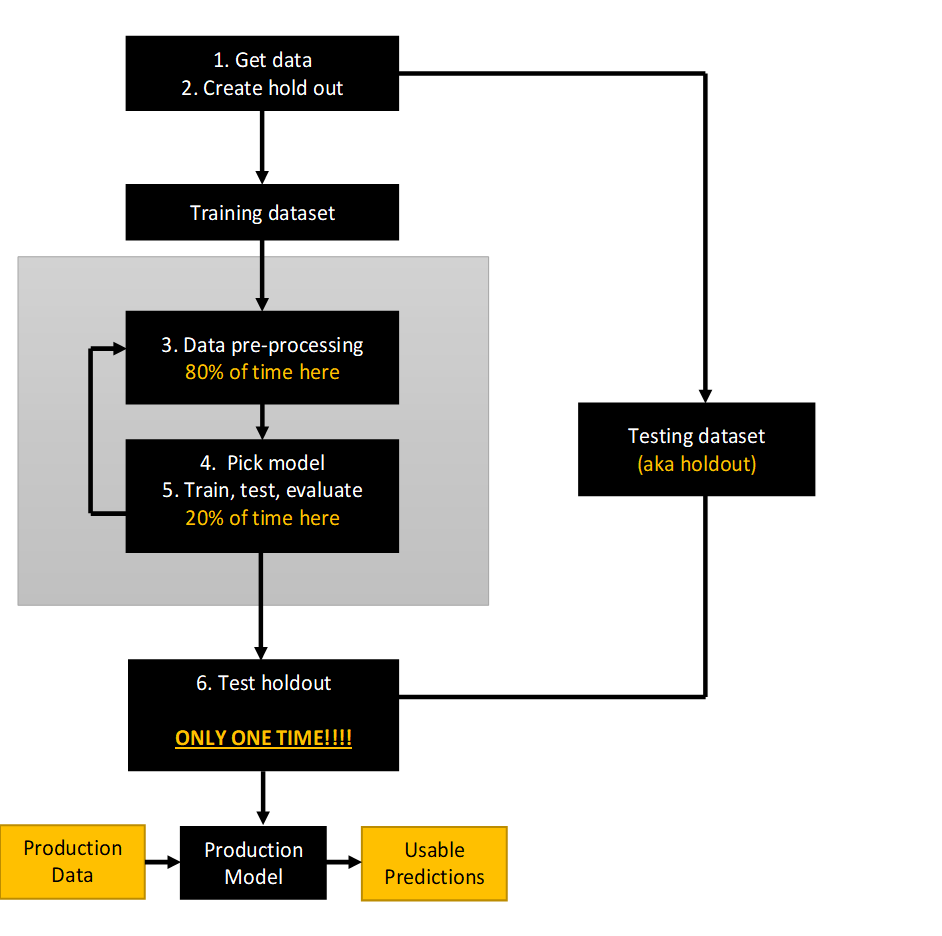# 5.3.5. Model selection¶You need to pick the right model for your job. One way to pick amongst the possible options is to consider whether or not you need a supervised model.

There is frankly FAR too much material to cover on the variety of models, and so many sites provide good introductions to the available options. Two starter links are below. Here, I’m just going to post the dichotomy of ML models from PythonDataScienceHandbook, which I think is a good starting point for understanding the ecosystem of models.

A dichotomy of ML models

Supervised learning models, try to predict “labels” (you can think of these as $$y$$ values) based on training data that already has the $$y$$ variable in it (“labeled data”). E.g.,

• Regression: Predicting continuous labels

• Classification: Predicting discrete categorical variables (two or more values)

Unsupervised learning models, build structure on unlabeled data. E.g.,

• Clustering: Models that detect and identify distinct groups in the data

• Dimensionality reduction: Models reduce the number of “features” (variables)

The PythonDataScienceHandbook has a nice introductory list of example applications for ML techniques, and the sklearn example page has a rather comprehensive set of examples with code!